# Motion problem

From Levíc to Košíc go car at speed 81 km/h.
From Košíc to Levíc go another car at speed 69 km/h.

How many minutes before the meeting will be cars 27 km away?

Result

t =  10.8 min

#### Solution:

$v_{ 1 } = 81 \ km/h \ \\ v_{ 2 } = 69 \ km/h \ \\ s = 27 \ km \ \\ \ \\ v = v_{ 1 }+v_{ 2 } = 81+69 = 150 \ km/h \ \\ t_{ 1 } = \dfrac{ s }{ v } = \dfrac{ 27 }{ 150 } = \dfrac{ 9 }{ 50 } = 0.18 \ h \ \\ t = t_{ 1 } \rightarrow h = t_{ 1 } \cdot \ 60 \ h = 10.8 \ h = \dfrac{ 54 }{ 5 } = 10.8 \ \text{ min }$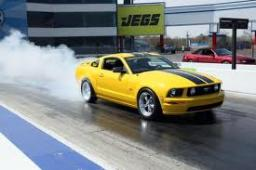Our examples were largely sent or created by pupils and students themselves. Therefore, we would be pleased if you could send us any errors you found, spelling mistakes, or rephasing the example. Thank you!

Leave us a comment of this math problem and its solution (i.e. if it is still somewhat unclear...):Be the first to comment!Tips to related online calculators
Need help calculate sum, simplify or multiply fractions? Try our fraction calculator.
Check out our ratio calculator.
Do you have a linear equation or system of equations and looking for its solution? Or do you have quadratic equation?
Do you want to convert length units?
Do you want to convert velocity (speed) units?
Do you want to convert time units like minutes to seconds?

## Next similar math problems:

1. Two portsFrom port A on the river, the steamer started at an average speed of 12 km/h towards port B. Two hours later, another steamer departed from A at an average speed of 20 km/h. Both ships arrived in B at the same time. What is the distance between ports A and
2. Where and when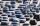The truck left Kremnica at 11:00 h at a speed of 60km/h. At 12:30 h, the passenger car started at an average speed of 80km/h. How many kilometers from Kremnica will the passenger car reach truck, and when?
3. Two shipsTwo ships sailed from Venice - a cargo speed of 10 knots and a ferry speed of 18 knots. At what time will the ferry catch up with the cargo ship if it sailed 10 hours later?
4. Two trains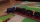Two trains departed from City A and City B against each other. They met after some time. The first train then took 9 hours to reach city B, and the second train took 4 hours to reach city A. In what proportion were the train speeds?
5. Cyclists and walkersA group of tourists started at 8:00 at speed 4 km/h walk. At half-past ten, another group started on a bike and caught up with a group of tourists at 10:50. What was the average speed of cyclists?
6. The escalator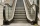I run up the escalator at a constant speed in the direction of the stairs and write down the number of steps A we climbed. Then we turn around and run it at the same constant speed in the opposite direction and write down the number of steps B that I climb
7. Bike rideMarek rode a bike ride. In an hour, John followed him on the same route by car, at an average speed of 72 km/h, and in 20 minutes he drove him. Will he determine the length of the way that Marek took before John caught up with him, and at what speed did M
8. Cheetah vs antelope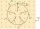When the cheetah began chasing the antelope, the distance between them was 120 meters. Although the antelope was running at 72km/h, the cheetah caught up with it in 12 seconds. What speed was the cheetah running?
9. The touristThe tourist traveled 78km in 3 hours. Part of the journey went on foot at 6km/h, the rest of the trip by bus at 30km/h. How long did he walk?
10. The bridge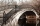A vehicle weighing 5,800 kg passes 41 km/h on an arched bridge with a radius of curvature of 62 m. What force is pushing the car onto the bridge as it passes through the center? What is the maximum speed it can cross over the center of the bridge so that
11. Propeller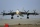The aircraft propeller rotates at an angular speed of 200 rad/s. A) What is the speed at the tip of the propeller if its distance from the axis of rotation is 1.5 m? B) What path does the aircraft travel during one revolution of the propeller at a spee
12. Skid friction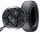Find the smallest coefficient of skid friction between the car tires and the road so that the car can drive at a 200 m radius at 108 km/h and does not skid.
13. Angled cyclist turnThe cyclist passes through a curve with a radius of 20 m at 25 km/h. How much angle does it have to bend from the vertical inward to the turn?
14. Two cylinders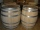Two cylinders are there one with oil and one with an empty oil cylinder has no fixed value assume infinitely. We are pumping out the oil into an empty cylinder having radius =1 cm height=3 cm rate of pumping oil is 9 cubic centimeters per sec and we are p
15. Positional energyWhat velocity in km/h must a body weighing 60 kg have for its kinetic energy to be the same as its positional energy at the height 50 m?
16. Doug bikedDoug biked 5 1/4 miles in 3/4 of an hour. What is his average speed?
17. A cold front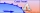At 1:00 PM, the temperature was at 46 degrees. Then, a cold front moved in and decreased the temperature 12 degrees per hour. The temperature at 6:00 PM = _____ degrees.